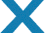We are committed to providing fast, efficient, and affordable software solutions that set new standards in the software development industry.# Pattern Example II

<?xml version="1.0" encoding="utf-8"?>

<!-- A pattern section. The  pattern name is AVI File LIST -->

<template name="AVI File LIST">

<!-- A template signature section. Alignment is 1. -->

<signature align="1">

<!-- A 4-byte signature at offset 0x00. -->

<field offset="0x00">4C 49 53 54</field> <!-- ANSI: LIST -->

</signature>

<!-- A data section. Its name is AVI File LIST . This is the main data section. It is not shown in the parsing tree as a section (its name is ignored).-->

< section name="AVI File LIST">

<!-- The first 4 bytes are read and shown as an ANSI  string. -->

<field type="char" size="4" name="Signature: LIST"/>

<!-- The current position is moved to the beginning of the file (4 bytes backward). -->

<goto offset="-4"/>

<!-- The first 4 bytes in the file are read and shown as an unsigned integer. The internal variable signature gets the value of the field. -->

<field type="uint32" name="Signature LIST as unsigned integer" var="signature"/>

<!-- A test against the condition ( signature == LIST ) -->

<if test="signature == 1414744396"> <!-- ANSI: LIST -->

<!-- The next 4 bytes are read and shown as an unsigned integer. The internal variable listSize gets its value. -->

<field type="uint32" name="Size of the data in the list" var="listSize"/>

<setvar var="endOfList" expr="offset + listSize"/>

<field type="char" size="4" name="List type"/>

<goto offset="-4"/>

<field type="uint32" base="hex" name="List type as unsigned integer in hex format" var="listType"/>

<!-- A test against condition ( listType == movi ) -->

<if test="listType == 0x69766f6d"> <!-- ANSI: movi -->

<!-- This chunk is not to be parsed. The current position is moved to the end of the chunk. -->

</if>

<setvar var="mediaType" expr="0"/>

<repeat test="endOfList > offset - 8">

<section name="CHUNK">

<field type="char" size="4" name="Chunk ID"/>

<goto offset="-4"/>

<field type="uint32" name="Chunk ID as unsigned integer" var="chunkId"/>

<if test="chunkId == 1414744396"> <!-- ANSI: LIST -->

<goto offset="-4"/>

<field type="uint32" base="hex" name="LIST chunk ID as unsigned integer in hex format" as-offset="start_position + offset - 4" assigned-template="AVI File LIST"/>

</if>

<field type="uint32" name="Size of the data in the chunk" var="chunkSize"/>

<setvar var="endOfChunk" expr="offset + chunkSize"/>

<!-- Rounding the value of the variable endOfChunk to a number divisible by 2. -->

<if test="endOfChunk &amp; 1">

<setvar var="endOfChunk" expr="endOfChunk &amp; 0xFFFFFFFE"/>

<setvar var="endOfChunk" expr="endOfChunk + 2"/>

</if>

<if test="chunkId == 1751742049"> <!-- ANSI: avih -->

<field type="uint32" name="Number of microseconds between frames"/>

<field type="uint32" name="Approximate maximum data rate of the file"/>

<field type="uint32" name="Alignment for data, in bytes"/>

<field type="uint32" name="Bitwise combination of zero or more of the flags"/>

<field type="uint32" name="Total number of frames of data in the file"/>

<field type="uint32" name="Initial frame for interleaved files"/>

<field type="uint32" name="Number of streams in the file"/>

<field type="uint32" name="Suggested buffer size for reading the file"/>

<field type="uint32" name="Width of the AVI file in pixels"/>

<field type="uint32" name="Height of the AVI file in pixels"/>

<field type="binary" size="16" name="Reserved"/>

</if>

<if test="chunkId == 1852994675"> <!-- ANSI: strn -->

<field type="char" size="chunkSize" name="Stream name"/>

</if>

<if test="chunkId == 1752331379"> <!-- ANSI: strh -->

<field type="char" size="4" name="Type"/>

<goto offset="-4"/>

<field type="uint32" base="hex" name="Type as unsigned integer in hex format" var="type"/>

<setvar var="mediaType" expr="type"/>

<field type="char" size="4" name="Handler (codec)"/>

<field type="uint32" base="hex" name="Flags"/>

<field type="uint32" name="Number of the first block of the stream that is present in the file"/>

<field type="uint32" name="Scale"/>

<field type="uint32" name="Rate"/>

<field type="uint32" name="Start time of stream"/>

<field type="uint32" name="Size of stream in units as defined in Rate and Scale"/>

<field type="uint32" name="Size of buffer necessary to store blocks of that stream"/>

<field type="uint32" name="Quality"/>

<field type="uint32" name="Sample size (number of bytes of one stream atom)"/>

</if>

<if test="chunkId == 1718776947"> <!-- ANSI: strf -->

<if test="mediaType == 0x73646976"> <!-- ANSI: vids -->

<field type="uint32" name="Number of bytes required by the structure"/>

<field type="int32" name="Width of the bitmap, in pixels"/>

<field type="int32" name="Height of the bitmap, in pixels"/>

<field type="uint16" name="Number of planes for the target device"/>

<field type="uint16" name="Number of bits-per-pixel"/>

<field type="uint32" name="Type of compression for a compressed bottom-up bitmap"/>

<field type="uint32" name="Size, in bytes, of the image"/>

<field type="int32" name="Horizontal resolution, in pixels-per-meter, of the target device for the bitmap"/>

<field type="int32" name="Vertical resolution, in pixels-per-meter, of the target device for the bitmap"/>

<field type="uint32" name="Number of color indexes in the color table that are actually used by the bitmap"/>

<field type="uint32" name="Number of color indexes that are required for displaying the bitmap"/>

</section>

</if>

<if test="mediaType == 0x73647561"> <!-- ANSI: auds -->

<section name="WAVEFORMATEX">

<field type="uint16" name="Waveform-audio format type"/>

<field type="uint16" name="Number of channels in the waveform-audio data"/>

<field type="uint32" name="Sample rate, in samples per second (hertz)"/>

<field type="uint32" name="Required average data-transfer rate, in bytes per second, for the format tag"/>

<field type="uint16" name="Block alignment, in bytes"/>

<field type="uint16" name="Bits per sample for the Waveform-audio format type"/>

<field type="uint16" name="Size, in bytes, of extra format information appended to the end of the WAVEFORMATEX structure"/>

</section>

</if>

</if>

</section>

</repeat>

</if>

<if test="signature != 1414744396">

<section name="Invalid LIST signature found">

</section>

</if>

</section>

</template>